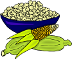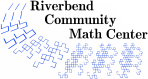# Fermi Questions

### Description

What would it take to fill this room with popcorn? How many people in the world are talking on their cell phones at this instant? If everyone in the country needed to be inoculated against a virulent strain of influenza, how quickly could this be done? Find the answers to these and other seemingly inaccessible questions by estimating and using knowledge gained from everyday experiences.

### Levels

• Grades K through 12.
• Adults will find this activity interesting.
• This activity can be extended to an undergraduate research project.

5 to 15 minutes

### Activity Time

Usually 1 to 4 hours per Fermi question

### Topics

• modeling
• estimation
• measurement
• algebraic expressions
• formulas
• problem solving

### Goals

• Identify and evaluate modeling strategies
• Generate many potential solutions to a given problem
• Apply principles and generalizations to new problems and situations
• Analyze problems from different points of view
• Make decisions as part of a group
• Communicate mathematical ideas verbally and in writing
• Work with a variety of measurement tools
• Create and utilize formulas to tackle a problem
• Use internet search tools to find information
• Hone estimation skills and confidence

### Materials

• journal or paper for recording findings
• pencils
• copies of handouts (optional)
• appropriate measurement tools (optional)
• appropriate equipment for gathering experimental evidence (optional)

### Prerequisites

• familiarity with basic measurement skills
• familiarity with common uses of addition, subtraction, multiplication, and division
• familiarity with fractions, decimals, and percents

### Credits

Sample Fermi questions were gleaned from the following sources

• Teachers and students from the greater South Bend, Indiana area
• Fermi Questions created by Louisiana Lessons in collaboration with the Math Forum
• Fermi Questions created for a Science Olympics competition by the Department of Physics at the University of Western Ontario

### Authors

Beth Marchant, Amanda Katharine Serenevy

### National Common Core Standards

 1.MD.2 Express the length of an object as a whole number of unit lengths by laying multiple copies of a shorter object end to end. 1.NBT.1 Count to 120, starting at any number less than 120. Read and write numerals and represent using objects. 1.NBT.2 Represent any number up to 100 using tens and ones (42 is four tens and two ones, or three tens and twelve ones, or two tens and twenty-two ones). 1.NBT.3 Compare two digit numbers and use >, =, < to record the results. 2.MD.1 Measure the length of an object by selecting and using appropriate tools such as rulers, yardsticks, meter sticks, and measuring tapes. 2.MD.2 Measure the length of an object twice, using length units of different lengths for the two measurements and describe how the measurements relate to the size of the unit chosen. 2.MD.3 Estimate lengths using units of inches, feet, centimeters, and meters. 2.NBT.1 Understand that the three digits of a three-digit number represent hundreds, tens, and ones. 2.NBT.2 Count within 1000; skip-count by 5s, 10s, and 100s. 2.NBT.3 Read and write numbers to 1000 using base-ten numerals, number names, and expanded form. 2.NBT.4 Compare two three-digit numbers based on meanings of the hundreds, tens, and ones digits, using >, =, and < symbols to record the results of comparisons. 2.NBT.7 Add and subtract within 1000, using concrete models or drawings and strategies and relate the method to a written method. 3.MD.5 Recognize area as an attribute of plane figures and understand concepts of area measurement. 3.MD.6 Measure areas by counting unit squares (square cm, square m, square in, square ft, and improvised units). 3.MD.7 Relate area to the operations of multiplication and addition (including the distributive property). 3.MD.8 Solve real world and mathematical problems involving perimeters of polygons, including finding the perimeter given the side lengths, finding an unknown side length, and exhibiting rectangles with the same perimeter and different areas or the same area and different perimeters. 3.NBT.1 Use place value understanding to round whole numbers to the nearest 10 or 100. 3.OA.1 Interpret products of whole numbers as equal groups of objects. 3.OA.2 Interpret whole-number quotients as objects partitioned into equal shares. 3.OA.3 Use multiplication and division within 100 to solve word problems in situations involving equal groups, arrays, and measurements quantities. 4.Focus.1 Develop understanding and fluency with multi-digit multiplication and division. 4.MD.2 Use the four operations to solve word problems involving distances, intervals of time, capacity, masses, and money, including problems involving simple fractions or decimals and problems that require use of simple unit conversions. 4.MD.3 Apply the area and perimeter formulas for rectangles in real world and mathematical problems. 5.Focus.2 Fluently add, subtract, multiply, and divide multi-digit numbers and make sense of standard algorithms with decimals using models for fractions and decimals. 5.Focus.3 Develop an understanding of volume. 5.MD.1 Convert among measurement units within a given measurement system. 5.MD.3 Recognize volume as an attribute of solid figures and understand the meaning of volume in terms of unit cubes. 5.MD.4 Measure volumes by counting unit cubes, using cubic cm, cubic in, cubic ft, and improvised units. 5.MD.5 Relate volume to the operations of multiplication and addition and solve real world problems involving volumes of rectangular prisms. 5.NBT.5 Fluently multiply multi-digit whole numbers using the standard algorithm. 5.OA.1 Use parentheses, brackets, or braces in numerical expressions and evaluate expressions with these symbols. 5.OA.2 Write simple expressions that record calculations with numbers, and interpret numerical expressions without evaluating them. 6.EE.2 Write, read, and evaluate expressions in which letters stand for numbers. 6.EE.4 Identify when two expressions are equivalent and understand what equivalence of expressions implies. 6.EE.6 Use variables to represent numbers and write expressions when solving real-world or mathematical problems. 6.Focus.1 Solve ratio and rate problems and understand related concepts. 6.Focus.2 Exhibit conceptual understanding and algorithmic fluency with rational numbers and the four operations. 6.Focus.3 Write, interpret, and use expressions and equations. 6.NS.2 Fluently divide multi-digit numbers using the standard algorithm. 6.NS.3 Fluently add, subtract, multiply, and divide multi-digit decimals using the standard algorithms. 6.RP.3 Use ratio and rate reasoning to solve real-world and mathematical problems (make tables, solve unit rate problems, work with percents, and convert measurement units). 7.EE.2 Understand that rewriting an expression in different forms in a problem context can shed light on the problem and how the quantities in it are related. 7.Focus.1 Develop understanding of and apply proportional relationships. 7.Focus.3 Solve problems involving scale drawings and informal geometric constructions, and solve problems involving surface area and volume. 7.G.4 Know the formulas for the area and circumference of a circle and use them to solve problems. 7.RP.2 Recognize and represent proportional relationships between quantities. 7.RP.3 Use proportional relationships to solve multi-step ratio and percent problems. 7.SP.2 Use data from a random sample to draw inferences about a population with an unknown characteristic of interest. 8.Focus.1 Formulate and reason about expressions and equations. 8.G.9 Know the formulas for the volumes of cones, cylinders, and spheres and use them to solve real-world and mathematical problems. HS.A-SSE.1 Interpret expressions that represent a quantity in terms of its context. HS.Modeling Modeling is the process of choosing and using appropriate mathematics and statistics to analyze empirical situations, to understand them better, and to improve decisions. Quantities and their relationships in physical, economic, public policy, social, and everyday situations can be modeled using mathematical and statistical methods. When making mathematical models, technology is valuable for varying assumptions, exploring consequences, and comparing predictions with data. HS.S-IC.1 Understand statistics as a process for making inferences about population parameters based on a random sample from that population. K.CC.1 Count to 100 by ones and tens. K.CC.2 Count forward from a given number instead of beginning at 1. K.CC.3 Write numbers from 0 to 20. Represent a number of objects with a written numeral. K.CC.4 Connect counting to cardinality. (Count objects and understand that numbers refer to quantities.) K.CC.5 Count up to 20 items arranged neatly and up to 10 scattered items. K.Focus.1 Represent, relate, and operate on whole numbers. K.MD.1 Describe several different measurable attributes of a single object (length, weight, capacity, temperature).
Riverbend Community Math Center
hello@riverbendmath.org
http://riverbendmath.org
(574) 339-9111This work placed into the public domain by the Riverbend Community Math Center.